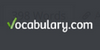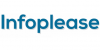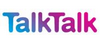### MultiplicationMultiplication (often denoted by the cross symbol `×`, or by the absence of symbol) is the third basic mathematical operation of arithmetic, the others being addition, subtraction and division (the division is the fourth one, because it requires multiplication to be defined). The multiplication of integers (including negative numbers), rational.....
Found on http://en.wikipedia.org/wiki/Multiplication

### multiplicationMultiplication is when a number can be added to itself a number of times. Multiplication is sometimes called repeated addition.
Example: 2 multiplied by 10 equals 20. (2 x 10 = 20)
See also: multiply
Found on http://www.bbc.co.uk/skillswise/glossary/

### MultiplicationSymbol : In arithmetic, multiplication of one number, a, by another, b, consists of adding a to itself b times. This kind of multiplication is commutative, i.e. . The identity element for arithmetic multiplication is 1, i.e. multiplication by 1 produces no change. In a series of multiplications the order in which they are carried out makes no diff...
Found on http://www.diracdelta.co.uk/science/source/m/u/multiplication/source.html

### Multiplication• (n.) The act or process of multiplying, or of increasing in number; the state of being multiplied; as, the multiplication of the human species by natural generation. • (n.) The art of increasing gold or silver by magic, -- attributed formerly to the alchemists. • (n.) An increase above the normal number of parts, especially of peta...
Found on http://thinkexist.com/dictionary/meaning/multiplication/

### multiplication(from the article `arithmetic`) ...that a repeated sum such as 5 + 5 + 5 is independent of the way in which the summands are grouped; it can be written 3 5. Thus, a second binary ... ...many systems, such as the real or complex numbers, there are other systems, such as the system of matrices o...
Found on http://www.britannica.com/eb/a-z/m/137

### multiplication1. The act or process of multiplying, or of increasing in number; the state of being multiplied; as, the multiplication of the human species by natural generation. 'The increase and multiplication of the world.' (Thackeray) ... 2. <mathematics> The process of repeating, or adding to itself, any given number or quantity a certain number of tim...
Found on http://www.encyclo.co.uk/local/20973

### multiplicationtimes noun an arithmetic operation that is the inverse of division; the product of two numbers is computed; `the multiplication of four by three gives twelve`; `four times three equals twelve`
Found on https://www.encyclo.co.uk/local/20974

### Multiplication[alchemy] Multiplication is the process in Western alchemy used to increase the potency of the philosopher`s stone, elixir or projection powder. It occurs near the end of the magnum opus in order to increase the gains in the subsequent projection. George Ripley gives the following definition of multiplication: Multiplication was also used t...
Found on http://en.wikipedia.org/wiki/Multiplication_(alchemy)

### Multiplication[music] The mathematical operations of multiplication have several applications to music. Other than its application to the frequency ratios of intervals (e.g., Just intonation, and the twelfth root of two in equal temperament), it has been used in other ways for twelve-tone technique, and musical set theory. Additionally ring modulation is...
Found on http://en.wikipedia.org/wiki/Multiplication_(music)

### MultiplicationMul`ti·pli·ca'tion noun [ Latin multiplicatio : confer French multiplication . See Multiply .] 1. The act or process of multiplying, or of increasing in number; the state of being multiplied; as, the multiplication of the human species by natural generation. ...
Found on http://www.encyclo.co.uk/webster/M/114

### multiplicationarithmetic operation determining the product of two numbers
Found on https://www.vocabulary.com/lists/310894

### multiplicationmultiplication, fundamental operation in arithmetic and algebra. Multiplication by a whole number can be interpreted as successive addition. For example, a number N multiplied by 3 is N + N + N. In general, multiplying positive numbers N and M gives the area of the rectangle with sides N and M. The ...
Found on http://www.infoplease.com/ce6/sci/A0834412.html

### multiplicationOne of the four basic operations of arithmetic, usually written in the form a × b or ab, and involving repeated addition in the sense that a is added to itself b times. Multiplication obeys the associative law (for example, a ×...
Found on http://www.talktalk.co.uk/reference/encyclopaedia/hutchinson/m0035997.html

### multiplicationThe operation that, for positive integers, consists of adding a number (the multiplicand) to itself a certain number of times. The operation is extended to other real numbers according to the rules governing the multiplicational properties of positive integers.
Found on http://www.daviddarling.info/encyclopedia/M/multiplication.html

### multiplication[n] - a multiplicative increase 2. [n] - an arithmetic operation that is the inverse of division
Found on http://www.webdictionary.co.uk/definition.php?query=multiplication
No exact match found